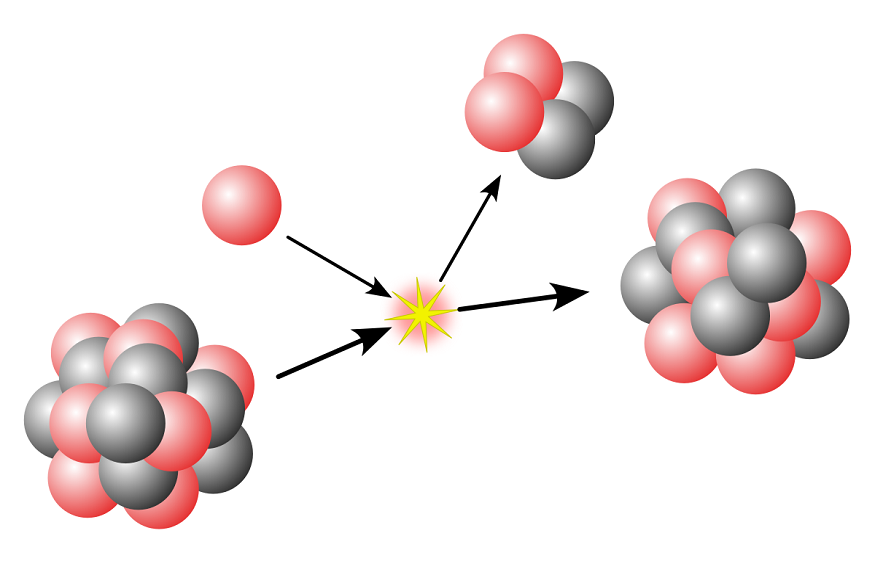460 Views

Radiation is one of the forms of energy. In the year 1896, Henry Becquerel discovered that the nucleus of an unstable atom loses its energy by emitting radiation. The property exhibited by certain matter in which emission of energy and subatomic particles takes place spontaneously is known as Radioactivity. Radioactivity is one such phenomenon that is used in various fields like agriculture, geology, archaeology, space, and even in the production of electricity. In the medical field, radioactive materials are used to kill cancerous tissue.

Henry Becquerel observed that in a drawer that had photographic plates, a small amount of uranium compound was wrapped in black paper and was kept in it. When the plates were examined, it was found that they were exposed! This phenomenon came to be known as radioactive decay. Radioactive decay is also referred to as nuclear decay, radioactive disintegration, radioactivity, and nuclear disintegration. Alpha decay, Beta Decay, and Gamma Decay are the types of radioactive decay. In the process of radioactive decay, an unstable atomic nucleus loses its energy through the emission of radiation. A material is said to be radioactive since it contains unstable nuclei.

We know that radioactive decay includes alpha decay, beta decay, and gamma decay. According to the radioactive decay law, it states that “The probability per unit time that a nucleus will decay is a constant, independent of time”.

### Alpha Decay

Alpha decay was first observed by Ernest Rutherford, who used alpha particles in his gold foil scattering experiment. Alpha decay is a type of radioactive decay where an unstable nucleus changes to another element by emitting particles like protons and neutrons. The ejected particle in this process is referred to as an alpha particle and is simply a helium nucleus. The emitted alpha particles are positively charged and have greater mass.

### Beta Decay

Beta decay is a type of radioactive decay in which a proton is transformed into a neutron or vice versa. This reaction takes place inside the nucleus of the radioactive sample. A beta particle is often referred to as an electron, but it can also be a positron.

There are two beta decay types: beta minus and beta plus decay.

### Gamma Decay

The type of radioactivity where unstable atomic nuclei consume more energy by an electromagnetic process is known as gamma decay. Gamma decay is also known as gamma emission. In this process, the nucleus with orbiting electrons have some energy, and when an electron jumps from a level of high energy to a level of low energy, there is an emission of a photon.